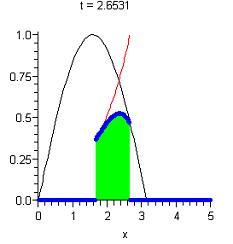Classroom Tips & Techniques: A First Look at Convolution - Maple Application Center
Application Center Applications Classroom Tips & Techniques: A First Look at Convolution

## Classroom Tips & Techniques: A First Look at ConvolutionThis Application runs in Maple. Don't have Maple? No problem!

This document summarizes a presentation of the concept of convolution in the Laplace transform portion of an introductory course in differential equations. The conceptual content of the presentation centers on the definition of the convolution integral. An animation is used to provide an intuitive understanding of what the convolution integral does, and two computational devices are presented for evaluating the convolution. For elementary functions such as e^(-t) and sin(t), the convolution integral is easily evaluated in Maple. After all, it's just a definite integral. For piecewise-defined functions, the convolution integral contains entangled conditional statements that easily become too complex for even Maple to unravel unaided. In this case, we show how to interpret the integration process in the framework of integration. Alternatively, we show that use of the convolution theorem for Laplace transforms leads to an immediate evaluation of the convolution for functions amenable to Maple's laplace and invlaplace commands.

#### Application Details

Publish Date: November 23, 2005
Created In: Maple 10
Language: English

#### Share

This application is in the following Collection:

calculus

5